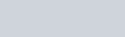Lorem ipsum dolor sit amet, consectetur adipiscing elit, sed do eiusmod tempor incididunt ut labore.

15 St Margarets, NY 10033
(+381) 11 123 4567
ouroffice@aware.com

### KMF#### How to Wrap Text in HTML Canvas

maxWidth && n > 0) {
// Then the line is finished, push the current line into “lineArray”
lineArray.push([line, x, y]);
// Increase the line height, so a new line is started
y += lineHeight;
// Update line and test line to use this word as the first word on the next line
line = `\${words[n]} `;
testLine = `\${words[n]} `;
}
else {
// If the test line is still less than the max width, then add the word to the current line
line += `\${words[n]} `;
}
// If we never reach the full max width, then there is only one line.. so push it into the lineArray so we return something
if(n === words.length – 1) {
lineArray.push([line, x, y]);
}
}
// Return the line array
return lineArray;
}

let grd = ctx.createLinearGradient(0, 1200, 800, 0);
ctx.fillStyle = grd;
ctx.fillRect(0, 0, 1200, 800);

// More text
ctx.font=”700 95px Helvetica”;
ctx.fillStyle=”white”;
let wrappedText = wrapText(ctx, “This line is way too long. It’s going to overflow – but it should line break.”, 85, 200, 1050, 140);
wrappedText.forEach(function(item) {
ctx.fillText(item, item, item);
})” data-lang=”text/javascript”>

``````let canvas = document.getElementById('canvas');
let ctx = canvas.getContext('2d');

canvas.width = 1200;
canvas.height = 800;

// @description: wrapText wraps HTML canvas text onto a canvas of fixed width
// @param ctx - the context for the canvas we want to wrap text on
// @param text - the text we want to wrap.
// @param x - the X starting point of the text on the canvas.
// @param y - the Y starting point of the text on the canvas.
// @param maxWidth - the width at which we want line breaks to begin - i.e. the maximum width of the canvas.
// @param lineHeight - the height of each line, so we can space them below each other.
// @returns an array of [ lineText, x, y ] for all lines
const wrapText = function(ctx, text, x, y, maxWidth, lineHeight) {
// First, start by splitting all of our text into words, but splitting it into an array split by spaces
let words = text.split(' ');
let line=""; // This will store the text of the current line
let testLine=""; // This will store the text when we add a word, to test if it's too long
let lineArray = []; // This is an array of lines, which the function will return

// Lets iterate over each word
for(var n = 0; n < words.length; n++) {
// Create a test line, and measure it..
testLine += `\${words[n]} `;
let metrics = ctx.measureText(testLine);
let testWidth = metrics.width;
// If the width of this test line is more than the max width
if (testWidth > maxWidth && n > 0) {
// Then the line is finished, push the current line into "lineArray"
lineArray.push([line, x, y]);
// Increase the line height, so a new line is started
y += lineHeight;
// Update line and test line to use this word as the first word on the next line
line = `\${words[n]} `;
testLine = `\${words[n]} `;
}
else {
// If the test line is still less than the max width, then add the word to the current line
line += `\${words[n]} `;
}
// If we never reach the full max width, then there is only one line.. so push it into the lineArray so we return something
if(n === words.length - 1) {
lineArray.push([line, x, y]);
}
}
// Return the line array
return lineArray;
}

let grd = ctx.createLinearGradient(0, 1200, 800, 0);
ctx.fillStyle = grd;
ctx.fillRect(0, 0, 1200, 800);

// More text
ctx.font="700 95px Helvetica";
ctx.fillStyle="white";
let wrappedText = wrapText(ctx, "This line is way too long. It's going to overflow - but it should line break.", 85, 200, 1050, 140);
wrappedText.forEach(function(item) {
ctx.fillText(item, item, item);
})``````